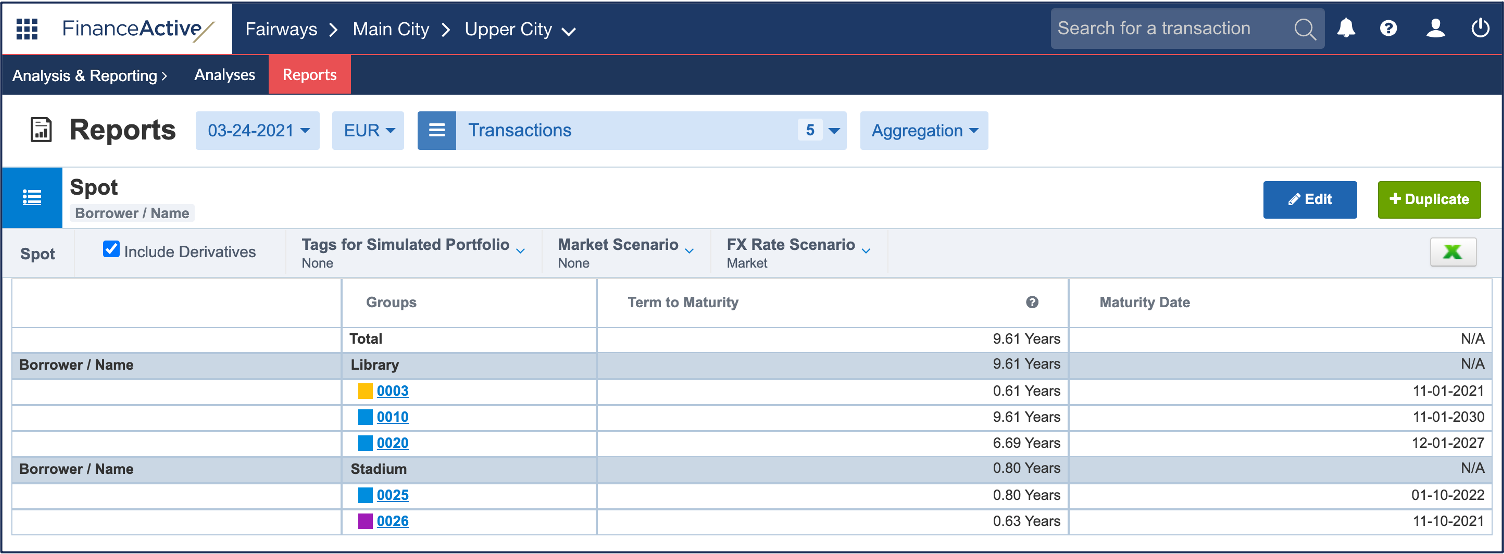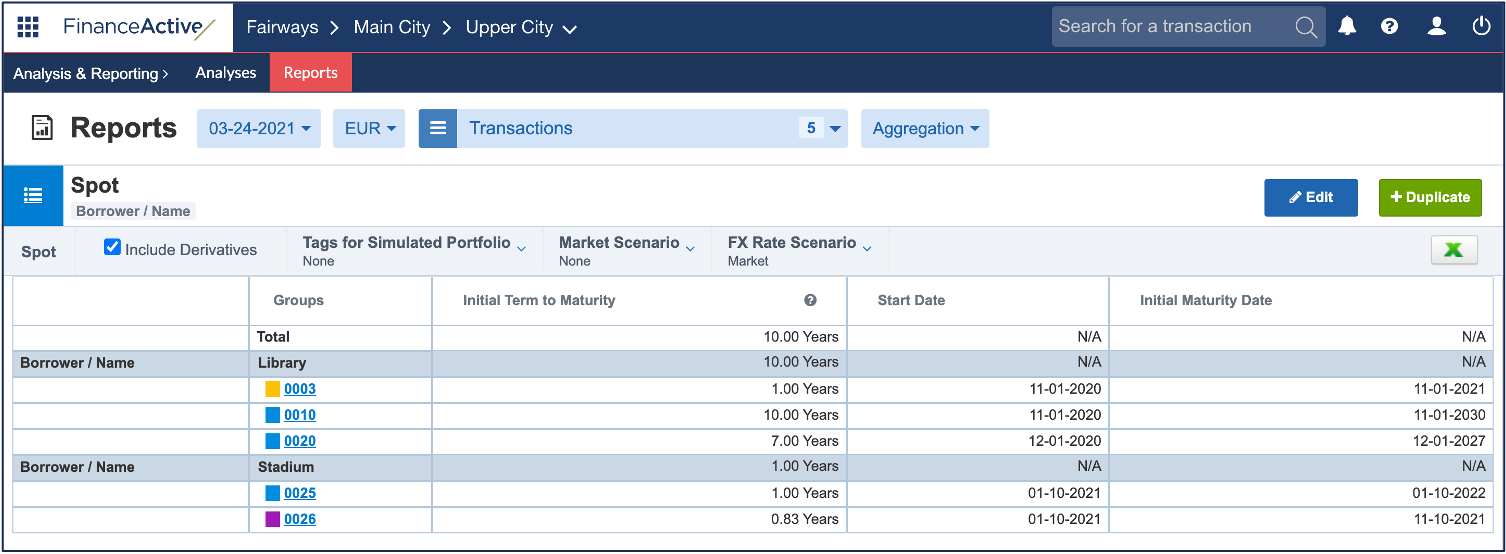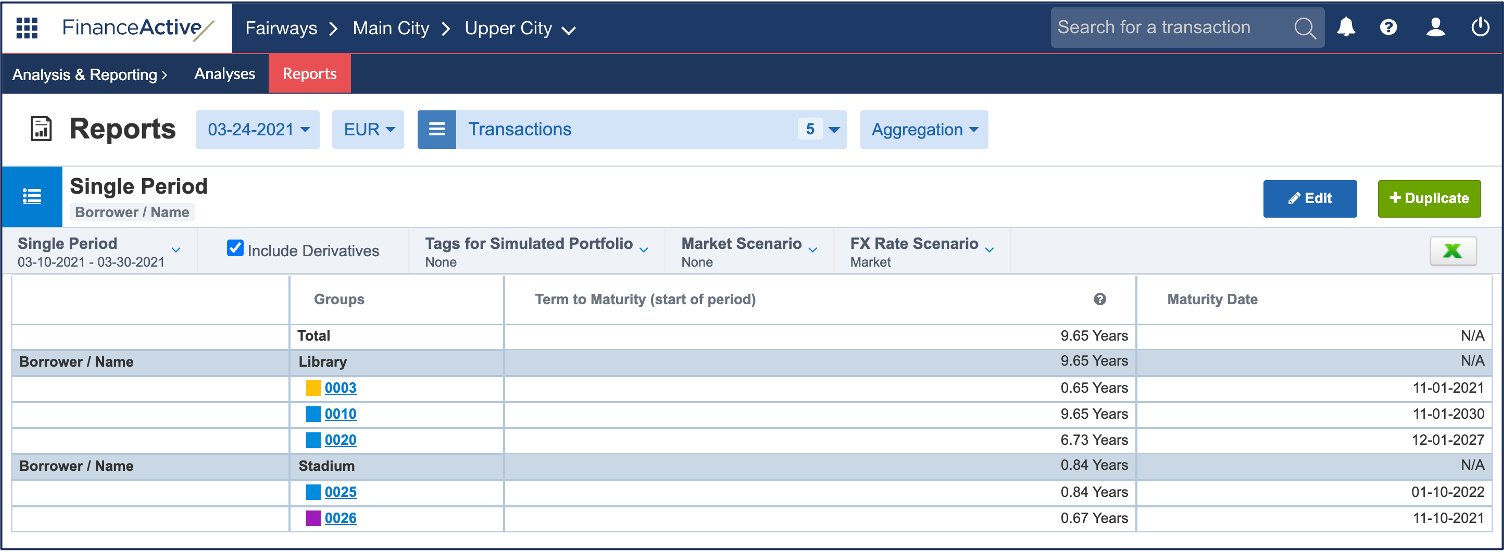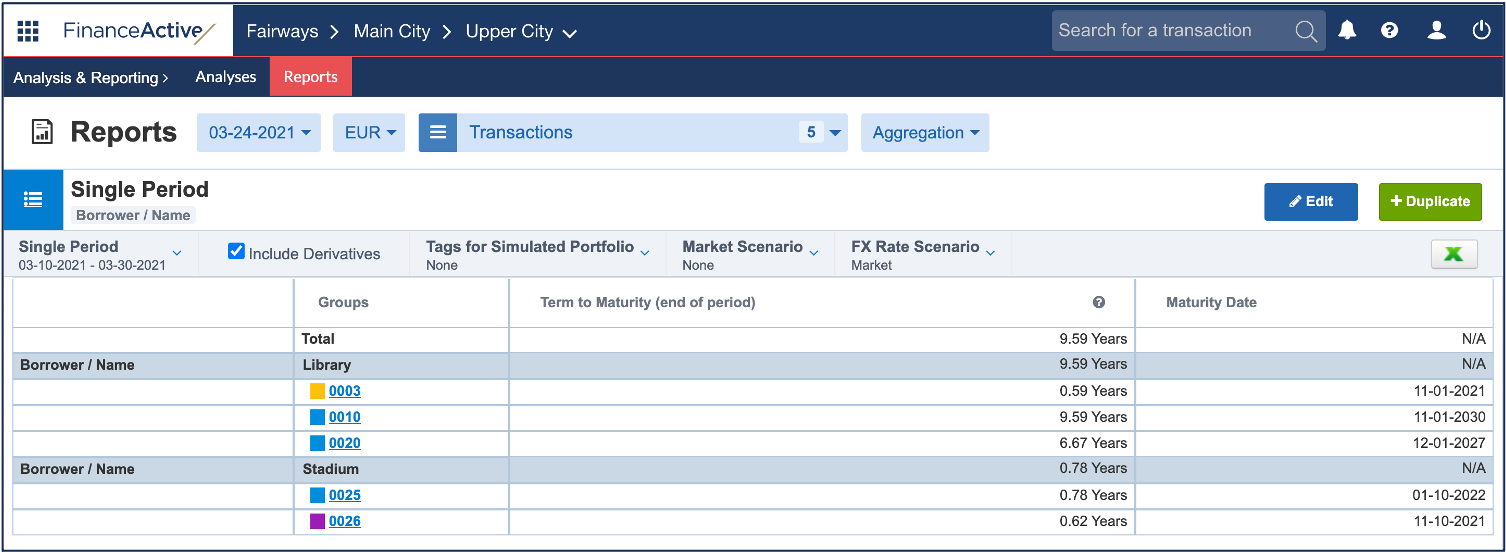# Term to Maturity

In Fairways Debt, the term to maturity is the remaining time till the transaction maturity date.

Note: The term to maturity of a group is the highest term to maturity displayed in the group.

## Spot Reports

Term to Maturity

Time from the report date to the transaction maturity date in years:

Count of days from the report date to the transaction maturity date / 365,25

In this example, the term to maturity of 0003 is 0,61 year, i.e. there is 0,61 year left from 24 March 2021 to 1 November 2021:

= Count of days from the report date to the transaction maturity date / 365,25

= 222 / 365,25

= 0,61• The highest term to maturity of Library is 9,61 years with 0010.
• The highest term to maturity of Stadium is 0,80 year with 0025.
• The highest term to maturity of all transactions displayed is 9,61 years with 0010.

Initial Term to Maturity

Time from the transaction start date to the initial maturity date of the transaction:

Count of days from the transaction start date to the transaction initial maturity date / 365,25

In this example, the initial term to maturity of 0003 is 1 year, i.e. there is 1 year between 1 November 2020 to 1 November 2021:

= Count of days from the transaction start date to the transaction initial maturity date / 365,25

= 365 / 365,25

= 1• The highest initial term to maturity of Library is 10 years with 0010.
• The highest term to maturity of Stadium is 1 year with 0025.
• The highest term to maturity of all transactions displayed is 10 years with 0010.

## Periodic / Single Period Reports

Term to Maturity (start of period)

Time from the start date of the report period to the transaction maturity date:

Count of days from the report start date to the transaction maturity date / 365,25

In this example, the term to maturity of 0003 is 0,65 year, i.e. there is 0,65 year left from 10 March 2021 to 1 November 2021:

= Count of days from the report start date to the transaction maturity date / 365,25

= 236 / 365,25

= 0,65• The highest term to maturity of Library is 9,65 years with 0010.
• The highest term to maturity of Stadium is 0,84 year with 0025.
• The highest term to maturity of all transactions displayed is 9,65 years with 0010.

Term to Maturity (end of period)

Time from the end date of the report period to the transaction maturity date.

Count of days from the report end date to the transaction maturity date / 365,25

In this example, the term to maturity of 0003 is 0,59 year, i.e. there is 0,59 year left from 30 March 2021 to 1 November 2021:

= Count of days from the report end date to the transaction maturity date / 365,25

= 216 / 365,25

= 0,59• The highest term to maturity of Library is 9,59 years with 0010.
• The highest term to maturity of Stadium is 0,78 year with 0025.
• The highest term to maturity of all transactions displayed is 9,59 years with 0010.